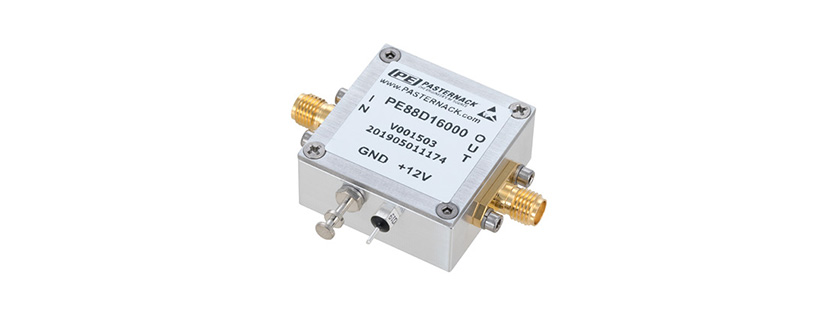# Difference Between Frequency Dividers and Power Dividers

Though both often dubbed as “dividers”, frequency dividers and power dividers perform very different functions. Where a power divider splits an input signal power to two or more output ports, a frequency divider actually outputs a frequency that is a fraction of the input frequency. A frequency divider should not be confused with a diplexer or frequency multiplexer, which divides an input signal into outputs dependent on the frequency content of the input signal. Moreover, a frequency divider should also not be confused with a duplexer, which is a 3-port device that enables two-way communication over a single antenna and feed line while providing isolation between the transmitter and receiver.

A power divider is a multi-port device, with a single port on one side and several ports on the other. Though power dividers do have frequency dependent operation, in their specified bandwidth they typically divide the power from the input port evenly to the output ports. There are power divider variants that do have asymmetric power dividing and have some ports that gain more of the input signal power than others, though these devices are typically known as couplers.

For an ideal two output power divider, the two output signals will be 3dB lower than the input signal. For an equally ideal four output power divider the output signals will each be 6dB lower than the input signal. In reality, power dividers suffer from insertion loss, VSWR, and exhibit imperfect isolation. The result of these degredations mean that not all of the input signal energy reaches the output ports of a power divider. Passive power divider architectures, such as the Wilkinson power divider can also double as a power combiner, as it is a symmetric passive device.

Frequency dividers on the other hand generate an output signal that is a fraction of the input signal in terms of frequency. These devices are also known as prescalers, or prescaler modules. A frequency divider is typically identified using it’s divide by ratio, such as a divide by 10 prescaler. RF frequency dividers are similar in function to digital dividers, but are constructed using analog components, such as mixers, filters, and amplifiers. This type of frequency divider is known as a regenerative frequency divider. The bandwidth of a regenerative frequency divider is determined by the mixer, filter, and amplifier used in its construction. The basic operation of a regenerative frequency divider is that the mixer feeds a low pass filter which feeds an amplifier that feeds both the output and a feedback line back to the mixer. The result of mixing the input signal and the mixed signal is the production of sum and difference frequencies of which the high frequency products are then filtered out by the low pass filter. As frequency dividers are active devices they require DC input power and do not operate symmetrically.

Learn more about Pasternack’s extensive line of RF Frequency Dividers and Power Dividers.

Courtesy of Pasternack

share post: